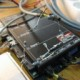Feeds:
Posts

## Screw Transformation

I am writing this while waiting for my “Robot Analysis” class at 11 am. Any of you who has basic knowledge about robotics, especially the kinematics must has ever heard the word Denavit-Hartenberg transformation. Well, I am not going to write about this method, but the other method which was AFAIK found the latest around 1971, if I am not mistaken.

I am not going to explain the whole theory, I will just show you the homework and Matlab program that I wrote for this transformation type. In Screw transformation, the reference and final point is arbitrarily chosen, this makes this method different to DH method.

Ok. Here’s the question:First we find transformation matrix using screw method. The screw axes location of Scorbot manipulator is shown in Table 1, then we define the reference, target position and then we can find the transformation matrix. With this transformation matrix we can derive the inverse kinematics.

a) Reference Position

 Table 1. Screw Axis Location of Scorbot Manipulator Joint i Si Soi 1 (0, 0, 1) (0, 0, 0) 2 (0, -1, 0) (a1, 0, 0) 3 (0, -1, 0) (a1 + a2, 0, 0) 4 (0, -1, 0) (a1 + a2 + a3, 0, 0) 5 (1, 0, 0) (0, 0, 0)

u0 = [0, 0, 1]T, v0 = [0, -1, 0]T, w0 = [1, 0, 0]T

and p0 = [a1 + a2 + a3, 0, 0] T

b) Target Position

u = [ux, uy, uz]T, v = [ux, uy, uz]T, w0 = [wx, wy, wz]T

and p = [px, py, pz]T

c) Transformation Matrix

By using Matlab® (See the attached code) the transformation matrices are found (Use the matlab code)

d) Inverse Kinematics

You can derive the inverse kinematics using the direct kinematics matrices calculated using the Matlab program, not that difficult, so I will leave this solution to you.

Matlab(R) 2007a source code:

```%%%%%%%%%%%%%%%%%%%%%%%%%%%%%%%%%%%%%%%%%%%%%%%%%%%%%%%%%%%%%%%%%%%%%
% Homework chapter 2 Number 11
% Book: Robot Analysis
% Name: Bondhan Novandy
% Student ID: 2007214012
%
% Description:  This file describe the screw tranformation, the input is the
%               screw axis location coordinate Si and Soi (table), the
%               output is the transformation matrix
%
% Dependency :  None
% Note       :  Tested on Matlab(R) 2007a
%
%%%%%%%%%%%%%%%%%%%%%%%%%%%%%%%%%%%%%%%%%%%%%%%%%%%%%%%%%%%%%%%%%%%%%

function [output] = screw_transform(val_sx, val_sy, val_sz, val_sox, val_soy, val_soz, symTheta,  joint_type)

global REVOLUTE PRISMATIC
REVOLUTE  = 0;
PRISMATIC = 1;

% Declare the symbolic variables
syms a11 a12 a13 a14 a21 a22 a23 a24 a31 a32 a33 a34 a41 a42 a43 a44
syms Theta
syms sx sy sz t
syms sox soy soz

sx = val_sx;
sy = val_sy;
sz = val_sz;
sox = val_sox;
soy = val_soy;
soz = val_soz;

Theta = 0;

Theta = symTheta;

if joint_type == REVOLUTE
t = 0;
% angle is not zero
elseif joint_type == PRISMATIC
% Theta is 0 then Sin(0) = 0 and Cos(0) = 1
Theta = 0;
% t is not zero
else
error('Joint type is wrong! REVOLUTE = 0 and PRISMATIC = 1');
end

% Formula according to 2.132
% Rotation matrix
a11 = (sx^2 - 1) * (1 - cos(Theta)) + 1;
a12 = sx * sy * (1 - cos(Theta)) - (sz * sin(Theta));
a13 = sx * sz * (1 - cos(Theta)) + (sy * sin(Theta));

a21 = sy * sx * (1 - cos(Theta)) + (sz * sin(Theta));
a22 = (sy^2 - 1) * (1 - cos(Theta)) + 1;
a23 = sy * sz * (1 - cos(Theta)) - (sx * sin(Theta));

a31 = sz * sx * (1 - cos(Theta)) - (sy * sin(Theta));
a32 = sz * sy * (1 - cos(Theta)) + (sx * sin(Theta));
a33 = (sz^2 - 1) * (1 - cos(Theta)) + 1;

a41 = 0;
a42 = 0;
a43 = 0;

% Translation Matrix
a14 = (t * sx) - (sox * (a11 - 1)) - (soy * a12) - (soz * a13);
a24 = (t * sy) - (sox * a21) - (soy * (a22 - 1)) - (soz * a23);
a34 = (t * sz) - (sox * a31) - (soy * a32) - (soz * (a33 - 1));
a44 = 1;

% Transform matrix
output = [a11 a12 a13 a14; a21 a22 a23 a24; a31 a32 a33 a34; a41 a42 a43 a44];

```
1. on November 14, 2007 at 1:01 am | ReplyNorm K
2. on November 14, 2007 at 9:18 am | ReplyBondhan Novandy